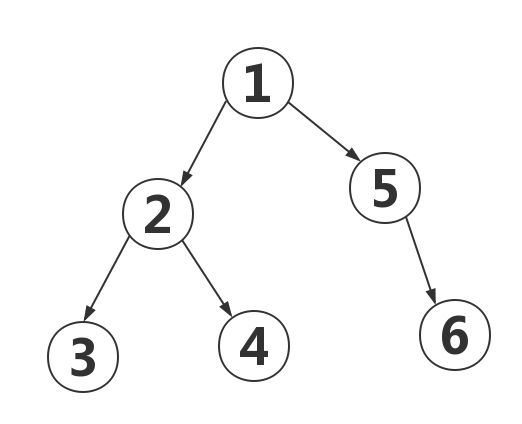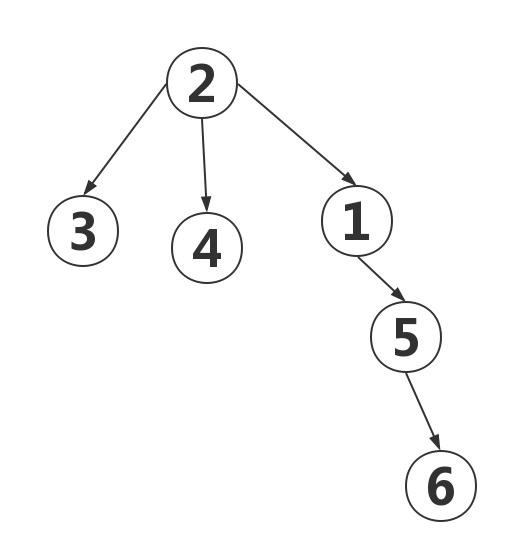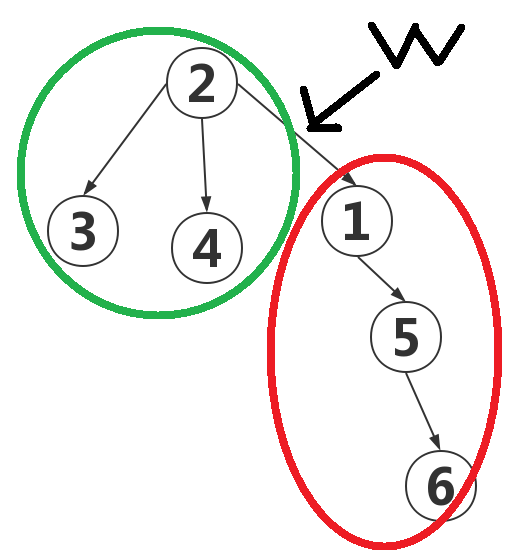• jzoj 2922 打印Hello word 众所周知，c++是许多人跨进代码世界的第一扇大门。但是你如何检验你的综合能力呢，有两个很好的网站它们分别是JZOJ与openjudge。我们先来讲OJ（JZOJ）上的题目。Tip：想要网址的我放在下面...
jzoj 2922 打印Hello word
众所周知，c++是许多人跨进代码世界的第一扇大门。但是你如何检验你的综合能力呢，有两个很好的网站它们分别是JZOJ与openjudge。我们先来讲OJ（JZOJ）上的题目。Tip：想要网址的我放在下面👇 JZOJ：www.jzoj.cn openjudge:openjudge.cnTip：openjudge推荐加入NOI。
现在进入正题，jzoj导图第一题：2922【入门】hello world!这一题主要讲了一个程序猿的入门 打印Hello world。 题目描述：输出“hello world!”；!也需要输出。 输入：无。 输出：hello world！ 总结一下这一题的坑：要有空格，大小写，符号。 那好下面代码献上
#include<bits/stdc++.h>
using namespace std;
int main(){
cout<<"hello world!"<<endl;
return 0;
}

初学者对这个头文件会有混淆，所以这里使用的是一个c++中的万能头。 代码就没什么含金量了，有一个重要的一点就是换行！！！
作者制作不易请勿不禁作者允许转载，谢谢配合（＞人＜；）
展开全文c++
• JZOJ 7045. 2021.04.05【2021省赛模拟】数学考试 题目大意 给出nnn个三次函数，其中xix_ixi​为第iii个函数的自变量，取值为[li,ri][l_i,r_i][li​,ri​]，另还需满足形如xa≤xb+dx_a\le x_b+dxa​≤xb​+d的mmm个...
JZOJ 7045. 2021.04.05【2021省赛模拟】数学考试
题目大意
给出

n

n

个三次函数，其中

x

i

x_i

为第

i

i

个函数的自变量，取值为

[

l

i

,

r

i

]

[l_i,r_i]

，另还需满足形如

x

a

≤

x

b

+

d

x_a\le x_b+d

的

m

m

个限制，求

∑

f

i

(

x

i

)

\sum f_i(x_i)

的最大值。

n

,

∣

l

i

∣

,

∣

r

i

∣

≤

100

，

m

≤

500

n,|l_i|,|r_i|\le 100，m\le 500

题解
限制特别难处理，一般会考虑建图跑网络流。本题采用的是求最小割。每个函数从

l

i

l_i

到

r

i

r_i

依次从源点到汇点连一条链，表示

p

(

p

∈

[

l

i

,

r

i

]

)

p(p\in[l_i,r_i])

的点连出的边权为

−

f

i

(

p

)

-f_i(p)

，断一条边表示该函数所取的自变量，则此处限制了自变量的取值。对每个限制，移项变为

x

b

≥

x

a

−

d

x_b\ge x_a-d

，即每取一个

x

a

x_a

，则需保证

x

b

≥

x

a

−

d

x_b\ge x_a-d

，那么从

a

a

的每个点

x

a

x_a

向

b

b

中表示

x

a

−

d

x_a-d

的点连边，边权为

i

n

f

inf

。其含义为若

a

a

的自变量为

x

a

x_a

，则必须

b

b

函数

x

a

−

d

x_a-d

以后取自变量（断一条边）。为了保证边权为正，给所有边都加上一个大常数

C

C

，最后答案为最小割减去

n

∗

C

n*C

再取相反数。
代码
#include<cstdio>
#include<cstring>
#include<algorithm>
using namespace std;
#define N 110
#define M 510
#define R 210
#define ll long long
#define E 100000000
int last[N * R], nxt[R * (N + M) * 2], to[R * (N + M) * 2], len;
int cur[N * R], gap[N * R], dis[N * R];
int p[N][R], n, m, S, T, tot;
ll ls[R * (N + M) * 2];
struct {
int a, b, c, d, l, r;
}a[N];
int ct(int k, int i) {
return i * i * i * a[k].a + i * i * a[k].b + i * a[k].c + a[k].d;
}
void add(int x, int y, ll w) {
to[++len] = y;
nxt[len] = last[x];
ls[len] = w + E;
last[x] = len;

to[++len] = x;
nxt[len] = last[y];
ls[len] = 0;
last[y] = len;

}
ll dfs(int k, ll flow)
{
if(k == T) return flow;
ll have = 0;
for(int i = cur[k]; i; i = nxt[i])
{
cur[k] = i;
if(dis[to[i]] + 1 == dis[k] && ls[i])
{
ll now = dfs(to[i], min(flow - have, ls[i]));
have += now;
ls[i] -= now, ls[i ^ 1] += now;
if(flow == have) return have;
}
}
cur[k] = last[k];
gap[dis[k]]--;
if(!gap[dis[k]]) dis[S] = tot;
gap[++dis[k]]++;
return have;
}
int main() {
int tn;
scanf("%d", &tn);
while(tn--) {
int i, j, x, y, d;
memset(last, 0, sizeof(last));
len = 1;
scanf("%d%d", &n, &m);
tot = 2, S = 1, T = 2;
for(i = 1; i <= n; i++) {
scanf("%d%d%d%d%d%d", &a[i].a, &a[i].b, &a[i].c, &a[i].d, &a[i].l, &a[i].r);
for(j = a[i].l; j < a[i].r; j++) {
p[i][j + 100] = ++tot;
add(tot, tot + 1, -ct(i, j));
}
p[i][a[i].r + 100] = ++tot;
}

for(i = 1; i <= m; i++) {
scanf("%d%d%d", &x, &y, &d);
for(j = a[x].l; j <= a[x].r; j++) {
if(j - d <= a[y].l) continue;
if(j - d > a[y].r) add(p[x][j + 100], T, 1e12);
else add(p[x][j + 100], p[y][j + 100 - d], 1e12);
}
}

memset(gap, 0, sizeof(gap));
memset(cur, 0, sizeof(cur));
memset(dis, 0, sizeof(dis));
gap = tot;
ll sum = 0;
while(dis[S] < tot) sum += dfs(S, 1e15);
sum -= (ll)n * E;
if(sum >= 1e10) puts("mei ji ge");
else printf("%lld\n", -sum);
}
return 0;
}

自我小结
本以为是一道数学题，但后来才发现函数只是个幌子。这题”奇奇怪怪的限制就想到网络流“理应很自然，但意识还是略有欠缺。知道网络流后连边建图解决限制求答案都十分自然。
展开全文• 做一个加法运算器，算出N组加数的和。（0 ） 输入 N+1行，每行两个整数，以0 0 结束。 输出 N行，每行一个整数，即算出的和。 样例 输入 复制 3 5 8 1 3 8 0 0 输出 复制 8 9 11c++
• 前言 290卡成145，十分开心。 正题 T1：密码 大意 N个数乘起来 考试时 看起来十分简单的高精乘单精 解题思路 102410241024其实是1024102410^{24}高精乘高精了解一下，30分QAQ ...#def...
前言
290卡成145，十分开心。

正题

T1：密码
大意
N个数乘起来
考试时
看起来十分简单的高精乘单精
解题思路

1024

1024

$1024$其实是

1024

10

24

$10^{24}$高精乘高精了解一下，30分QAQ
代码(高精乘高精我就不解释了吧)
#include<cstdio>
#include<cstring>
#define M 2500
using namespace std;
long long a[M+1],n,k[M],b[M+1],l,lo;
{
memset(k,0,sizeof(k));
char c;
scanf("%s",c);
l=strlen(c);
for (int i=1;i<=l;i++)
k[i]=c[l-i]-48;
}
{
for (int i=1;i<=lo;i++)
{
for (int j=1;j<=l;j++)
{
b[i+j-1]+=a[i]*k[j];
b[i+j]+=b[i+j-1]/10;
b[i+j-1]%=10;
}
}
lo=-1;
for (int i=M;i>=1;i--)
{
if (b[i]!=0&&lo==-1) lo=i;
a[i]=b[i];b[i]=0;
}
}
void write()
{
int w=M;
while (w>1&&!a[w]) w--;
int flag=w;
for (;w;w--)
printf("%d",a[w]);
}
int main()
{
scanf("%d",&n);
a=1;lo=1;
for (int i=1;i<=n;i++)
{
}
write();
}

T2：宝石
大意
有一个

m∗m

m

∗

m

$m*m$的矩阵，然后

n

n

$n$个宝石价值不同在矩阵里，然后一个为k∗k$k\ast k$$k*k$的东西要求碰到大宝石价值最大。
考试时
敲了一个矩阵前缀和然后

O(n2)

O

(

n

2

)

$O(n^2)$暴力枚举拿了60分
解题思路
定义一个长度m，高度为k的扫描线，然后在线内的用线段树维护最大长度为k的字段和。
代码
#include<cstdio>
#include<algorithm>
using namespace std;
struct treenode{
int l,r,w,lazy;
}a;
struct node{
int x,y,w;
}d;
int m,n,k,maxs;
void build(int k,int l,int r)
{
a[k].l=l;a[k].r=r;
if (l==r) return;
int mid=(l+r)/2;
build(k*2,l,mid);
build(k*2+1,mid+1,r);
}
void ddata(int k)
{
a[k*2].w+=a[k].lazy;
a[k*2+1].w+=a[k].lazy;
a[k*2].lazy+=a[k].lazy;
a[k*2+1].lazy+=a[k].lazy;
a[k].lazy=0;
}
void updata(int l,int r,int k,int num)
{
if (a[k].l==l&&a[k].r==r)
{
a[k].w+=num;
a[k].lazy+=num;
return;
}
ddata(k);
if (a[k*2].r>=r) updata(l,r,k*2,num);
else if (a[k*2+1].l<=l) updata(l,r,k*2+1,num);
else updata(l,a[k*2].r,k*2,num),updata(a[k*2+1].l,r,k*2+1,num);
a[k].w=max(a[k*2].w,a[k*2+1].w);
}
//以上为线段树
bool cmp(node x,node y)
{
return x.y<y.y;
}
int main()
{
scanf("%d%d%d",&m,&n,&k);
for (int i=1;i<=n;i++)
{
scanf("%d%d%d",&d[i].x,&d[i].y,&d[i].w);
}
sort(d+1,d+1+n,cmp);//排序
build(1,1,m);//建树
int w=1;//扫描上限
for (int i=1;i<=n;i++)//枚举下限
{
while (d[i].y-d[w].y>k)//更新上限
{
updata(d[w].x,min(d[w].x+k,m),1,-d[w].w);//去掉
w++;
}
updata(d[i].x,min(d[i].x+k,m),1,d[i].w);//维护字段和
maxs=max(maxs,a.w);//查询最大字段和
}
printf("%d",maxs);
}

T3：页
大意
一个序列，每次取中间的放到头或尾，求至少多少次可以变为单调递增。
考试
刚开始打了个贪心，然后发现数据不是很大，然后打了一个广搜，为了防止超时打了一个卡时间的结果就炸了30。把卡时间的去掉后100
解题思路
广搜然后map库(或哈希表)去重
代码
#include<cstdio>
#include<map>
#include<string>
#include<iostream>
#include<queue>
#include<ctime>
using namespace std;
map<string,int> f;
string s,mb,state;
void bfs()
{
if (s==mb)
{
printf("0");
return;
}
state=s;f[s]=1;
do
{
for (int i=1;i<=n/2;i++)
swap(s[i],s[n/2+1]);//放在头
if (!f[s])
{
state[++tail]=s;
f[s]=k+1;
if (s==mb)//已经完成
{
printf("%d",k);
return;
}
}
for (int i=n;i>n/2+1;i--)
swap(s[i],s[n/2+1]);//放在尾
if (!f[s])
{
state[++tail]=s;
f[s]=k+1;
if (s==mb)//已经完成
{
printf("%d",k);
return;
}
}
}
}
int main()
{
scanf("%d",&n);
s+="*";mb+="*";
for (int i=1;i<=n;i++)
{
scanf("%d",&a[i]);
s+=i+48;
mb+=i+48;
}
for (int i=1;i<n;i++)
for (int j=i+1;j<=n;j++)
{
if (a[i]>a[j])
{
swap(a[i],a[j]);
swap(mb[i],mb[j]);//确定目标状态
}
}
bfs();
return 0;
}

T4：景点中心
大意
有n个景点构成一颗树，然后每个景点有不同数量的学生，边有不同的长度，定一个景点为中心要求所有学生到达这个点的路径长度和最小。
考试
敲出了正解，结果没注意范围30QAQ。
解题思路
一棵树然后就想到了树形dp，然后想起来有个东西叫二次扫描换根法，之后就推出了正解：
首先我们先以1为根进行一遍求出

f[i]

f

[

i

]

$f[i]$和

zn[i]

z

n

[

i

]

$zn[i]$，

f[i]

f

[

i

]

$f[i]$是表示点i子树的学生走到点i的路径长度和，然后

zn[i]

z

n

[

i

]

$zn[i]$表示点i的子树的学生人数总和。
然后我们就求出了以i为根节点的情况下的路径和，我们尝试推到第二层的节点。这是一颗以1为根节点的树，然后我们变为以2为根节点然后我们会发现图中绿色部分（原第二个节点的子树部分）都少走了一条路w，而图中红色部分（其余部分）都多走了一条路w，所以我们可以自己计算：

c[x]=c[father]+(zn−zn[x])∗w−zn[x]∗w

c

[

x

]

=

c

[

f

a

t

h

e

r

]

+

(

z

n

[

1

]

−

z

n

[

x

]

)

∗

w

−

z

n

[

x

]

∗

w

c[x]=c[father]+(zn-zn[x])*w-zn[x]*w
从而

O(1)

O

(

1

)

$O(1)$的时间复杂度内计算出下一个点为根时的距离和。

代码
#include<cstdio>
#include<algorithm>
#include<cstring>
#define N 1000001
using namespace std;
struct node{
int next,to,w;
}a[N];
int ls[N],tot,n,x,y,w,v[N];
long long c[N],f[N],zn[N],maxs,num[N];
{
a[++tot].to=y;a[tot].w=w;
a[tot].next=ls[x];ls[x]=tot;
}
void dp(int x,int dep)//第一次扫描
{
v[x]=true;
zn[x]=num[x];//记录人数
f[x]=num[x]*dep;//统计
for (int i=ls[x];i;i=a[i].next)
{
int y=a[i].to;
if (!v[y])
{
dp(y,dep+a[i].w);
f[x]+=f[y];
zn[x]+=zn[y];//累计
}
}
}
void zdp(int x,int dep,int fa,int from)//第二次扫描
{
v[x]=true;
if (x!=1)
c[x]=c[fa]+(zn-zn[x])*a[from].w-zn[x]*a[from].w;//计算
if (c[x]<c[maxs]) maxs=x;
for (int i=ls[x];i;i=a[i].next)
{
y=a[i].to;
if (!v[y])
zdp(y,dep+a[i].w,x,i);//计算子节点
}
}
int main()
{
freopen("data.txt","r",stdin);
scanf("%d",&n);
for (int i=1;i<=n;i++)
{
scanf("%lld",&num[i]);
}
for (int i=1;i<n;i++)
{
scanf("%d%d%d",&x,&y,&w);
}
c=1e18;
maxs=0;
dp(1,0);
memset(v,0,sizeof(v));
c=f;
zdp(1,0,0,0);
printf("%lld\n%lld",maxs,c[maxs]);
}

后续
zyc大佬太强了
                            m e
|
\ /
V
/ / ======  / /     orz orz orz orz orz orz orz orz orz
/ / ======  / /       orz orz orz orz orz orz orz orz orz
+ =========== +        orz orz orz orz orz orz orz orz orz
| [zyc dalao] |         orz orz orz orz orz orz orz orz orz
+ =========== +          orz orz orz orz orz orz orz orz orz
\ \    ====== \ \         orz orz orz orz orz orz orz orz orz
\ \   ======  \ \         orz orz orz orz orz orz orz orz orz
展开全文扫描线 线段树
• JZOJ 6997. 2021.03.06【2021省赛模拟】排列 题目大意 ppp为一个111到nnn的排列，令F(p)=∑i=1nmin⁡j=1iapi⨁bpjF(p)=\sum_{i=1}^n \min_{j=1}^i a_{p_i}\bigoplus b_{p_j}F(p)=∑i=1n​minj=1i​api​​⨁bpj​​...
JZOJ 6997. 2021.03.06【2021省赛模拟】排列
题目大意

p

p

为一个

1

1

到

n

n

的排列，令

F

(

p

)

=

∑

i

=

1

n

min

⁡

j

=

1

i

a

p

i

⨁

b

p

j

F(p)=\sum_{i=1}^n \min_{j=1}^i a_{p_i}\bigoplus b_{p_j}

，求使

F

(

p

)

F(p)

最小且字典序最小的

p

p

。

n

≤

50

n\le50

题解
若选择

b

y

b_y

和

a

x

a_x

构成一组贡献，则相当于从

y

y

往

x

x

连了一条边，即要求

y

y

在排列中需要在

x

x

的前面，把所有的

(

x

,

y

)

(x,y)

对应的边都连出来，边权为

b

y

⨁

a

x

b_y\bigoplus a_x

，则题目可以转化为在这些边中选出一些边构成树形结构，其中边的方向都由父亲指向儿子，并最小化边权和。基本上就是求最小树形图，但同时要考虑排列字典序最小。可以依次枚举每个位置填什么数，并固定下来，用剩下的点求最小树形图。每次选择权值和最小的且最小的数填入当前位置。在实现上有细节需要注意：最小树形图中，可能会出现自己连向自己的情况，并且也是合法的；用剩下的点求最小树形图时，根节点不仅是当前固定下来的点，而是前面已经固定好的所有点合并作为根。
代码
#include<cstdio>
#include<cstring>
#include<algorithm>
using namespace std;
#define N 110
int a[N], b[N], dis[N][N], ar[N], tot, n;
int ch[N], st[N], fa[N], vi[N], e[N][N], sn[N][N];
int solve(int x) {
int s = dis[fa[x]][x];
int ci[N];
ci = 0;
for(int i = 1; i <= tot; i++) if(sn[x][i]) ci[sn[x][i]] = i, ci++;
for(int i = 1; i <= ci; i++) fa[ci[i]] = ci[i % ci + 1], s += solve(ci[i]);
return s;
}
int count(int v0) {
int i, j, k, ok = 0, l = -1;
memset(sn, 0, sizeof(sn));
while(!ok) {
l++;
memset(fa, 0, sizeof(fa));
memset(vi, 0, sizeof(vi));
// 非根节点的贡献
for(i = 1; i <= e[l]; i++) if(e[l][i] != v0) {
int mi = 1e9, p, x = e[l][i];
// 在集合内，由非根、非自己转移
for(j = 1; j <= e[l]; j++) if(e[l][j] != x && dis[e[l][j]][x] < mi) mi = dis[e[l][j]][x], p = e[l][j];
fa[x] = p;
// 由自己、根或已确定的序列转移
int mii = (x <= n ? dis[x][x] : 1e9); p = x;
for(j = 1; j <= ar; j++) if(dis[ar[j]][x] < mii) mii = dis[ar[j]][x], p = ar[j];
if(mii <= mi) {
vi[x] = 1;
fa[x] = p;
}
}
// vi[] = 1 --> 挂入确定的点，不可能产生环。需计入下一层，可任意往后挂
vi[v0] = 1; int id = 1;
ok = 1;
for(i = 1; i <= e[l]; i++) if(vi[e[l][i]]) e[l + 1][++e[l + 1]] = e[l][i];
for(i = 1; i <= e[l]; i++) if(!vi[e[l][i]]) {
id++;
st = 0;
int x = e[l][i];
while(!vi[x]) {
vi[x] = id;
st[++st] = x;
x = fa[x];
}
if(vi[x] == id) {
//构成环，缩点
ok = 0;
tot++;
for(j = 1; j <= tot; j++) dis[tot][j] = dis[j][tot] = 1e9;
for(j = 1; st[j] != x && j <= st; j++);
int j0 = j;
for(j; j <= st; j++) {
int x = st[j];
sn[tot][x] = j - j0 + 1;
for(k = 1; k < tot; k++) {
dis[tot][k] = min(dis[tot][k], dis[x][k]);
dis[k][tot] = min(dis[k][tot], dis[k][x] - dis[fa[x]][x]);
}
}
e[l + 1][++e[l + 1]] = tot;
for(j = 1; st[j] != x && j <= st; j++) {
e[l + 1][++e[l + 1]] = st[j];
}
}
else {
//未构成环，加入下一层
for(j = 1; j <= st; j++) e[l + 1][++e[l + 1]] = st[j];
}
}
}
int s = 0;
for(i = 1; i <= e[l]; i++) {
int x = e[l][i];
s += solve(x);
}
return s;
}
int main() {
int i, j, k;
scanf("%d", &n);
for(i = 1; i <= n; i++) scanf("%d", &a[i]);
for(i = 1; i <= n; i++) scanf("%d", &b[i]);
for(i = 1; i <= n; i++)
for(j = 1; j <= n; j++) dis[i][j] = b[i] ^ a[j];
for(i = 1; i <= n; i++) {
int mi = 1e9, x;
ar++;
for(j = 1; j <= n; j++) if(!ch[j]) {
memset(e, 0, sizeof(e));
for(k = 1; k <= n; k++) if(!ch[k]) e[++e] = k;//未选加入集合
tot = n;
ar[i] = j;
int t = count(j);
// 当前根的贡献
int mii = 1e9;
for(k = 1; k <= i; k++) mii = min(mii, dis[ar[k]][j]);
if(t + mii < mi) mi = t + mii, x = j;
}
ch[x] = 1;
ar[i] = x;
if(i == 1) printf("%d\n", mi);
}
for(i = 1; i <= n; i++) printf("%d ", ar[i]);
return 0;
}

自我小结
这题看似是最小树形图的模板题，其实却有很多与之不同且极其需要注意的地方，同时是第一次写最小树形图，思路和细节上都出现了许多问题。
展开全文最小树形图
• JZOJ 6868. 【2020.11.17提高组模拟】数树 题目大意 给出一棵大小为NNN的树，树边有向，求∀i∈[1,N)(ai,ai+1)∉Edge\forall i\in[1,N)(a_i,a_{i+1})\notin Edge∀i∈[1,N)(ai​,ai+1​)∈/​Edge的排列方案数。 N...树上背包
• 题目链接：https://jzoj.net/senior/#main/show/3302 阿狸和桃子居住的世界里, 只有一个国家, 这个国家有很多城市, 每个城市直接由中央政府管辖. 电力是这个国家的唯一能源, 但是每个城市的发电能力都不一样, 于是就...上下界费用流
• 题目链接:https://jzoj.net/senior/#contest/show/3008/2 题目大意 两个数(a,b)(a,b)(a,b)，两个操作 (a,b)−>(a,b+1)(a,b)->(a,b+1)(a,b)−>(a,b+1) (a,b)−>(a∗b,b)(a,b)->(a*b,b)(a,b)−>(a...搜索 dp
• JZOJ7月17日提高组T2 数组题目DescriptionInputOutputSample InputSample OutputData Constraint题解题意分析Code 题目 Description Input Output Sample Input 输入样例1： 3 2 7 5 4 2 输入样例2： 5 3 1 5 4 3...
• JZOJ 6974. 【2021.02.01冬令营模拟】联邦解体 题目大意 长为nnn的原序列中每个数有两个权值Ai,BiA_i,B_iAi​,Bi​，求保持相对顺序不变的前提下，把原序列分割为两个子序列后所有相邻两项的A,BA,BA,B权值之差的...DP
• JZOJ 6979. 【2021.02.03冬令营模拟】天各一方 题目大意 求nnn个点组成的所有不同连通图中，111到nnn的最短距离之和。 n≤400n\le400n≤400 题解 很关键的一点是，因为是所有连边的方案，所以111到nnn和111到222...
• 有X+Y+Z个三元组(x[i],y[i],z[i])，请你从每个三元组中挑数，并满足以下条件： 1、每个三元组中可以且仅可以选择一个数（即x[i],y[i],z[i]中的一个） 2、选择x[i]的三元组个数恰好为X 3、选择y[i]的三元组个数恰好为...
• JZOJ 11.21 提高B组反思 T1 第二类斯特林数 直接套公式 S(i,j)=S(i−1,j−1)+S(i−1,j)∗jS(i,j)=S(i-1,j-1)+S(i-1,j)*jS(i,j)=S(i−1,j−1)+S(i−1,j)∗j 由于过大， unsigned long longunsigned\ long\ ...
• 题目链接：https://jzoj.net/senior/#main/show/3086 moreDmoreDmoreD城的城市轨道交通建设终于全部竣工，由于前期规划周密，建成后的轨道交通网络由 2n2n2n条地铁线路构成，组成了一个nnn纵nnn横的交通网。如下图...最短路
• 4721--兰姐姐的愤怒 (jzoj.cn) 题目 4721 兰姐姐的愤怒 时间限制: 1.000 Sec内存限制: 128 MB 题目描述 兰姐姐是来自火星的女王。 由于兰姐姐实在太漂亮了，所以有许多仰慕她的人前来追求她。兰姐姐会给这个...c++
• JZOJ 6873. 【2020.11.19提高组模拟】飞翔的鸟 题解 n,kn,kn,k的数据范围看起来就知道要用矩乘，但障碍的位置是不确定的。 如果暴力枚举n−2n-2n−2次障碍的位置，分别计算每种情况障碍左右两边的方案数，再左右...矩阵乘法
• 有一棵n 个节点的树，初始时所有节点都为空。Alan 和Bob 在树上玩游戏，双方轮流进行，Alan先手。每轮中，Alan 可以选择一个空节点x，在x 上放一个波澜哥；Bob 每轮可以选择一个空节点y，将y 节点以及所有与y 相邻的...
• 题目 题解 –谁知道这道水题O(n^2)暴力有90分，当然是要动脑筋，我就不讲了 谁又知道这道题要用权值线段树维护，来拿最后那十分 （代码量 * 10 == 分数 * 10/9）QAQ ...首先权值线段树就是把原来存下标的 l 和 ...
• JZOJ 7047. 2021.04.07【2021省赛模拟】染色 题目大意 一个n∗mn*mn∗m的棋盘，一次染色可以把任何一行或任何一条左下-右上方向的对角线上所有格子都染黑，求各种染色方案可以得到的不同最终形态数。 n,m≤500n,m\...DP
• Description 因为小Y 是知名的白富美，所以自然也有很多的追求者，这一天这些追求者打算进行一次游戏来踢出一些人，小R 自然也参加了。 这个游戏有n 个人参加，每一轮随机选出一个还没有出局的人x，接着x 会出局。...
• } 链接 JZOJ链接 1290--【入门】行李托运费 (jzoj.cn) 车站行李托运费标准是：10千克或10千克以下，每千克收费1.5元，超过10千克的行李，按每超过1千克增加2.5元进行收费。试编一程序，输入行李的托运费，算出行李...c++
• 题目描述： 一家软件开发公司有两个项目，并且这两个项目都由相同数量的m个子项目组成，对于同一个项目，每个子项目都是相互独立且工作量相当的，并且一个项目必须在m个子项目全部完成后才算整个项目完成。...
• JZOJ 6493. 【GDOI2020模拟03.04】迷宫 题解 这道题的题面比较玄学，乍一看还以为是道PJ难度的最短路。。。 其实，题目的设定十分有趣，你被放进了迷宫里，只能分清门（A/B/C/D），而不能分清房间（0除外），也就是...状压DP
• 题目描述 小H与小Y刚刚参加完UOIP外卡组的初赛，就迫不及待的跑出考场对答案。 “吔，我的答案和你都不一样！”，小Y说道，”我们去找神犇们问答案吧”。 外卡组试卷中共有m道判断题，小H与小Y一共从其他n个神犇...
• 【NOIP2015模拟11.5】JZOJ8月5日提高组T3 旅行 题目 若不存在第kkk短路径时，输出“Stupid Mike” 题解 题意 给出一个有nnn个点的树 问这nnn个点两两之间经过道路为奇数的第kkk短的路径长度是多少 路径长度特别...
• 链接 题目描述 有A，B两个不相等的数，请将其中较大数打印出来。 输入 输入只有一行，包括2个整数。之间用一个空格分开。 输出 输出只有一行（这意味着末尾有一个回车符号），包括1个整数。...这一题就是让你比较两个数...
• 蛋糕 题目描述： 今天是Bessie的生日，他买了一个蛋糕和朋友们一起分享，蛋糕可以看成是一个R行C列的表格，共有R*C个格子，每个格子都有一个0至9的数字，表示该格子蛋糕拥有的巧克力。现在Bessie要把蛋糕横的切3刀再...
• 题目 你有n个水槽，分别装ai的水。 你可以选定一个区间，平均他们的水。 你可以进行上述操作任意次。 请最小化ai的字典序，并输出。 N<...一个很好的性质是，前缀和之后字典序大小关系不变。...using names...
• 正题 题目大意 nnn个物品，用空间换价值。mmm个方式，用价钱换空间。 要求价值超过p的情况下价钱最低。 解题思路 先算出超过ppp至少要多少空间。然后在算出这个空间至少需要多少价钱。...using namespace s...多重背包 dp...